Example Questions

Example Question #52 : Data Analysis

In a particular high school, 200 students are freshmen, 150 students are sophomores, 250 students are juniors, and 100 students are seniors. Twenty percent of freshmen are in honors classes, ten percent of sophomores are in honors classes, twelve percent of juniors are in honors classes, and thirty percent of seniors are in honors classes.

If a student is chosen at random, what is the probability that that student will be a student who attends honors classes?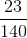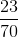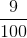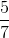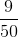Explanation:

First calculate the number of students: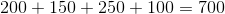The probability of drawing an honors student will then be the total number of honors students divided by the total number of students attending the school: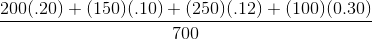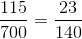Example Question #51 : Probability

In a particular high school, 200 students are freshmen, 150 students are sophomores, 250 students are juniors, and 100 students are seniors. Twenty percent of freshmen are in honors classes, ten percent of sophomores are in honors classes, twelve percent of juniors are in honors classes, and thirty percent of seniors are in honors classes.

If a student is chosen at random, what is the probability that that student will be a senior student and a student who does not attend honors classes?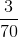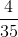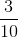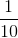Explanation:

First calculate the number of students:The percentage of seniors that do not attend honors classes is: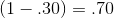Therefore, the probability of selecting a student who is a senior and one who does not attend honors classes is: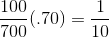Example Question #262 : Data Analysis

There is a bag of marbles that contains thre different color marbles, red, blue and green. There are 12 red, 15 blue marbles and 13 green marbles. There is also a coin with heads and tails that are equally likely. If a person were to randomly select a marble, while flipping a coin, what is the probability that a person will draw a blue marble and see tails on the coin flip?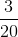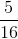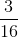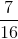Explanation:

The probability of selecting a blue marble is the number of blue marbles out of the total number of marbles: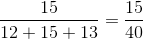The probability of seeing a tails is one half. Multiplying the probability of drawing a blue marble and seeing tails gives the probability of both events occurring.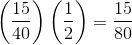The numerator and the demoninator have a common factor of 5 so that this fraction simplifies to:Example Question #101 : Probability

A drawer containswhite shirts,gray shirts, andblue shirts, and no other shirts. If shirts are seclected at random from the drawer, what is the probability that two shirts of the same color are selected?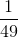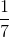Explanation:

The probability of choosing the first shirt of a certain color is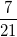.

The probability of choosing a second shirt of that color is now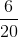because both there are nowshirts of that color left andshirts total left in the drawer.

So the probability of choosing both the first shirt AND the second shirt is*, which equals.

Example Question #101 : How To Find The Probability Of An Outcome

11 cards are placed into a box numbered 5 - 15. If one card is randomly drawn from the box, what is the probabiltiy that a prime number will be on the card?

1/2

6/11

2/5

4/11

3/5

4/11

Explanation:

Possible numbers 5,6,7,8,9,10,11,12,13,14,15 (11 total numbers)

Prime numbers are 5,7,11,13 (4 total prime numbers)

totalnumber of prime numbers/ total numbers in box

Example Question #265 : Data Analysis

Which of the following would not change the probability that a randomly-drawn card from a standard deck of 52 will be a king?

Removing the hearts from the deck, and adding...

...the aces of spades from thirteen other decks.

...the face cards and the joker from another deck.

...the twos from three other decks, plus the joker from a fourth deck.

...the aces, the twos, the threes, and the joker from another deck.

...the clubs from another deck.

...the clubs from another deck.

Explanation:

Removing the hearts from the deck reduces the number of kings in the deck by one. Since each of the five choices involves replacing the thirteen cards with thirteen different cards, thereby keeping the number of cards constant, we want the choice that restores the number of kings to four - that is, the choice that involves adding exactly one king. The only choice that involves this is the choice to add the clubs from another deck.

Example Question #266 : Data Analysis

Balls are placed in a box, each marked with a letter. One ball is marked with an "A"; two balls are marked with a "B"; three are marked with a "C", and so forth up to twenty-six with a "Z".

A ball is drawn at random. Give the probability that the selected ball will be marked with a vowel.

Note: for purposes of this problem, "Y" is a consonant.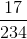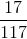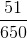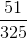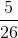Explanation:

The number of balls placed in the box will be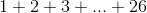The sum of the firstnatural numbers is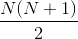so there are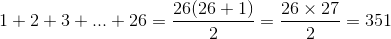,

balls in the box.

The five vowels are "A", "E", "I", "O", and "U", which are the first, fifth, ninth, fifteenth, and twenty-first letters of the alphabet. Consequently, there are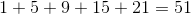balls marked with vowels, and the probability of drawing one of these balls is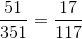.

Example Question #101 : How To Find The Probability Of An Outcome

Balls are placed in a box, each marked with a letter. One ball is marked with an "A"; two balls are marked with a "B"; three are marked with a "C", and so forth up to twenty-six with a "Z".

A ball is drawn at random. Give the probability that the selected ball will be marked with an "M".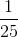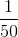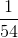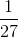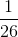Explanation:

The number of balls placed in the box will beThe sum of the firstnatural numbers isso there are,

balls in the box.

"M" is the thirteenth letter of the alphabet, so thirteen balls are marked with an "M". The probability of drawing this letter is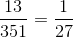Example Question #102 : How To Find The Probability Of An Outcome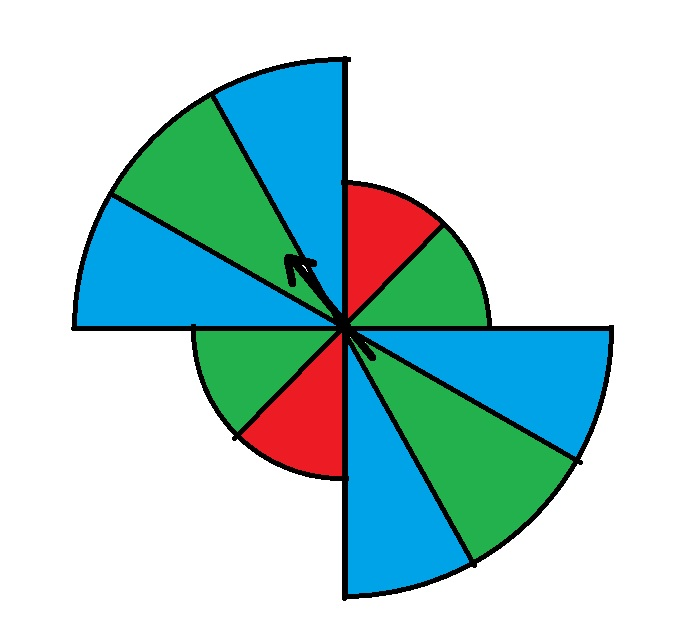In the above spinner, the larger sectors have radius twice that of the smaller sectors.

If the above spinner is spun, what are the odds against it stopping while pointing to a red sector?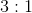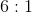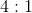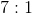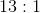Explanation:

The probability that the spinner will stop in a red sector depends on the angles of the different colored sectors, not on the sizes of the sectors. The radii of the sectots is therefore irrelevant.

Each red region is half of a quarter-circle, so the two sectors are, together, one fourth of a circle. The odds against the spinner stopping in a red circle are therefore three fourths to one fourth, or 3 to 1, against.

Example Question #103 : How To Find The Probability Of An Outcome

The ace of spades and the ace of clubs are taken out of a standard 52-card deck. They are replaced by the king of spades and the king of clubs from another deck.

Let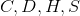be the probabilities that a randomly drawn card is a club, diamond, heart, and spade, respectively. What happened to each probability when the switch was made?and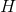remained the same;and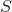decreased.andincreased;andremained the same.

All four probabilities remained the same.andremained the same;andincreased.anddecreased;andremained the same.